NCERT Solutions for Class 6 Maths Chapter 14 Practical Geometry Ex 14.1 are part of NCERT Solutions for Class 6 Maths. Here we have given NCERT Solutions for Class 6 Maths Chapter 14 Practical Geometry Ex 14.1.

 Board CBSE Textbook NCERT Class Class 6 Subject Maths Chapter Chapter 14 Chapter Name Practical Geometry Exercise Ex 14.1 Number of Questions Solved 5 Category NCERT Solutions

## NCERT Solutions for Class 6 Maths Chapter 14 Practical Geometry Ex 14.1

Question 1.
Draw a circle of radius 3.2 cm.
Solution :Steps of Construction

• Open the compasses for the required radius 3.2 cm, by putting the pointer on 0 and opening the pencil upto 3.2 cm.
• Draw a point with a sharp pencil and marks it as O in the centre.
• Place the pointer of the compasses where the centre has been marked.
• Turn the compasses slowly to draw the circle.

Question 2.
With the same centre O, draw two circles of radii 4 cm and 2.5 cm.
Solution :
Steps of Construction
1. For circle of radius 4 cm

• Open the compasses for the required radius 4 cm, by putting the pointer on 0 and opening the pencil upto 4 cm.
• Place the pointer of the compasses at O.
• Turn the compasses slowly to draw the circle.

2. For circle of radius 2.5 cm

• Open the compasses for the required radius 2.5 cm. by putting the pointer on 0 and opening the pencil upto 2.5 cm.
• Place the pointer of the compasses at O.
• Turn the compasses slowly to draw the circle.

Question 3.
Draw a circle and any two of its diameters. If you join the ends of these diameters, what is the figure obtained? What figure is obtained if the diameters are perpendicular to each other? How do you check your answer?
Solution :
(i) On joining the ends of any two diameters of the circle, the figure obtained is a rectangle.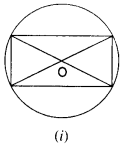(ii) On joining the ends of any two diameters of the circle, perpendicular to each other, the figure obtained is a square.
To check the answer, we measured the sides and angles of the figure obtained.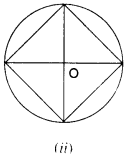Question 4.
Draw any circle and mark points A, B and C such that:
(a) A is on the circle.
(b) B is in the interior of the circle.
(c) C is in the exterior of the circle.
Solution :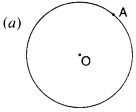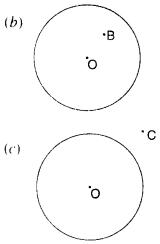Question 5.
Let A, B be the centres of two circles of equal radii; draw them so that each one of them passes We need a ruler here. through the centre of the other. Let them intersect at C and D. Examine whether $$\overline { AB }$$ and $$\overline { CD }$$ are at right angles.
Solution :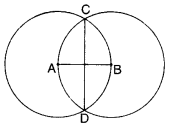Yes! $$\overline { AB }$$ and $$\overline { CD }$$ are at right angles.

We hope the NCERT Solutions for Class 6 Maths Chapter 14 Practical Geometry Ex 14.1 help you. If you have any query regarding NCERT Solutions for Class 6 Maths Chapter 14 Practical Geometry Ex 14.1, drop a comment below and we will get back to you at the earliest.